Time: 3 Hours                                                                                                     Max. Marks: 100

NOTE: There are 9 Questions in all.

·      Question 1 is compulsory and carries 20 marks. Answer to Q. 1. must be written in the space provided for it in the answer book supplied and nowhere else.

·      Out of the remaining EIGHT Questions answer any FIVE Questions. Each question carries 16 marks.

·      Any required data not explicitly given, may be suitably assumed and stated.

Q.1       Choose the correct or best alternative in the following:                                         (2x10)

a.       Consider a lossless line with characteristic impedance Ro and VSWR = S.  Then the impedance at the point of a voltage maxima equals

(A)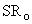(B)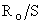(C)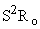(D)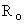b.      If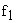and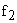are half power frequencies and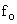is the resonance frequency, the selectivity of RLC circuit is given by

(A)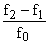(B)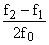(C)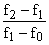(D)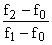c.   A symmetrical T network has characteristic impedance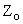and propagation constant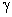.  Then the series element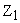and shunt element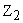are given by

(A)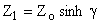and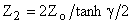(B)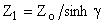and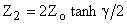(C)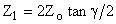and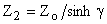(D)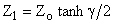and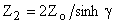d.   A function is given by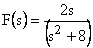.  It will have a finite zero at

(A)    Infinity                                         (B)  Anywhere on the s-plane

(C)  On the imaginary axis                   (D)  On the origin

e.   For a linear passive bilateral network

(A)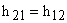(B)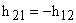(C)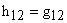(D)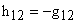f.    A constant K band-pass filter has pass-band from 1000 to 4000 Hz.  The resonance frequency of shunt and series arm is a

(A)     2500 Hz.                                     (B)  1500 Hz.

(C)  2000 Hz.                                      (D)  3000 Hz.

g.   A constant voltage source with 10V and series internal resistance of 100 ohm is equivalent to a current source of

(A)     100mA in parallel with 100 ohm.

(B)     1000mA in parallel with 100 ohm.

(C)     100V in parallel with 10-ohms.

(D)    100mA in parallel with 1000 ohm.

h.   Input impedance of a short-circuited lossless line with length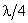is

(A)(B) zero

(C) infinity                                           (D)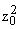i.    Laplace transform of unit impulse is

(A)   u(s)                                              (B) 1

(C) s                                                   (D) 1/s

j.    In a two terminal network, the open circuit voltage at the given terminal is 100V and the short circuit at the same terminal gives 5A current.  If a load of 80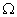resistance is connected at the terminal, the load current is given by

(A)  1 Amp                                         (B)  1.25 Amp

(C)  6 Amp                                         (D)  6.25 Amp

Answer any FIVE Questions out of EIGHT Questions.

Each question carries 16 marks.

Q.2     a.   Differentiate between unilateral elements and the bilateral elements.                     (4)

b.   A capacitor of 10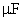capacitance is charged to a potential difference of 200 V and then connected in parallel with an uncharged capacitor of 30.  Calculate

(i)                  The potential difference across the parallel combination.

(ii)                Energy stored by each capacitor.                                                (4)

c.   An exponential voltage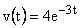is applied at time t = 0 to a series RLC circuit comprising of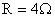, L = 1H and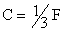.  Obtain the complete particular solution for current i(t).  Assume zero current through inductor L and zero charge across capacitance C before application of exponential voltage.                                                                       (8)

Q.3     a.   Find the Laplace transform of the functions:

(i)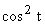.                                          (ii)  t sin 2t.                                             (4)

b.   Find the value of v(t) given its laplace transform V(s)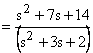.                 (4)

c.   Find the sinusoidal steady state solution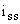for a series RC circuit.                      (8)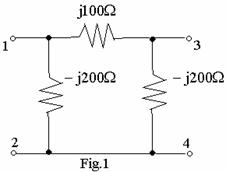Q.4     a.   A symmetrical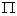section is given in Fig.1.  Find out its equivalent          T-network.                      (6)

b.   State initial value theorem in the Laplace transform .  What is the value of the function at t = 0, if its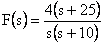(4)

c.   Determine the input impedance of a transmission line when the far end is:

(i)   Short circuited.                              (ii)  Open circuited.                                 (6)

Q.5     a.   State Norton’s theorem.  Derive the Thevenin equivalent from a given Norton equivalent circuit.                                                                 (8)

b.   In the network shown in Fig.2, find the value of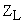, so that it draws the maximum power from the source.  Also determine the maximum power.    (8)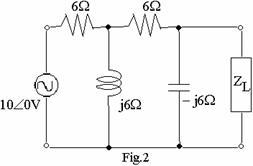Q.6     a.   Find the transmission parameters of the network shown in Fig.3.  Determine whether the given circuit is reciprocal and symmetric or not.          (8)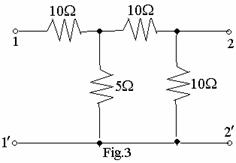b.   A transform voltage is given by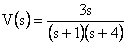.  Plot the pole zero plot in the s-plane and obtain the time domain response.                         (8)

Q.7     a.   A 100 mH inductor with 500self-resistance is in parallel with a 5nF capacitor.  Find the resonant frequency of the combination.  Find the impedance at resonance, quality factor of the circuit and the half power bandwidth.                                                                                            (10)

b.   With the help of frequency curves, explain the effect of coefficient of coupling on the primary and the secondary currents.                               (6)

Q.8     a.   Define the terms voltage standing wave ratio (VSWR) and reflection coefficient.  State and derive the relations between VSWR and reflection coefficient.                                                            (2+2+4)

b.   When the far end of the transmission line is open circuited, the input impedance is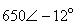ohms and when the line is short-circuited, the input impedance is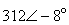ohms.  Find the characteristic impedance of the line.                                                                                                          (4)

c.   A lossless line has a characteristic resistance of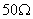.  The line length is 1.185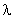.  The load impedance is 110 + j80.  Find the input impedance.                                                            (4)

Q.9     a.   What is an attenuator?  Give two uses of an attenuator.  With the help of a suitable example give the relation for the attenuation constant (N) in Nepers, for a symmetric T-network.                               (2+2+4)

b.   Design an m-derived T section (high pass) filter with a cut off frequency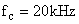,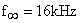and a design impedance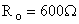.           (8)